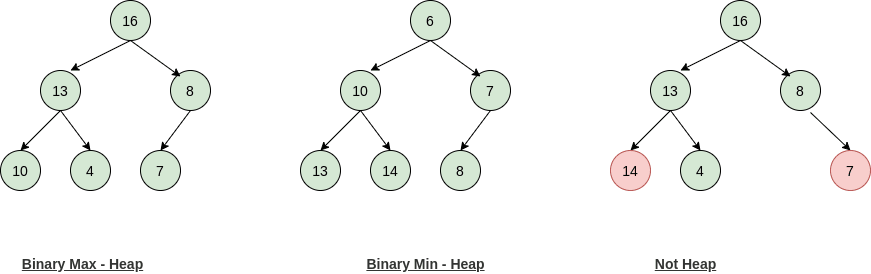Home Data Structures Binary Heap Data Structure

# Binary Heap Data Structure [Introduction]

A Binary Heap is a form of Binary Tree with the following additional properties –

1. A Binary Heap is a complete Binary tree with all levels except the last level is completely filled. The last level of the tree is not completely full and nodes at each level are filled from left to right.
2. A heap can be a Max-Heap or a Min-Heap. In Max-Heap the root node is the largest node and all nodes at a level will be strictly less than or equal to the parent node. In Max-Heap the root node is the smallest node and nodes at a level will be strictly greater than or equal to the parent node.

Example –#### Properties of a Binary Heap

1. The height of Binary Heap is always O(log N), where N is the number of nodes in a given Heap.
2. For a given height h, the maximum number of nodes in a heap will be  2h+1 -1 and the minimum number of nodes will be 2h
3. The total number of leaf nodes in a Heap will be (N+1)/2  where N is the number of nodes in a given heap.
4. The elements from (⌊N/2⌋ +1) to N are all leaf nodes.
5. The maximum number of nodes at a given height will be ⌈N/2h+1⌉ where h is the given height and N is the number of nodes in a heap.
6. A Binary Heap can be either a Max-Heap or a Min-Heap.

#### Representation of a Heap

A binary heap is generally represented as an array in following ways –

1. The root-node will be stored at arr
2. The left-child of an ith node will be stored at arr[i]
3. The right-child of an ith node will be stored at arr[i+1]

For a given node (say i) –

1. The parent will be stored at arr[(i-1)/2]
2. The left-child will be stored at arr[2*i + 1]
3. The right-child will be stored at arr[2*i + 2]

### The time complexity of various operations on a Binary Heap

###### Time Complexity
Heapify O(log N)
Search Node O(N)
Insert Node O(log N)
Delete Random Node O(log N)
Build Heap O(N)
HeapSort O(N*logN)

Want to suggest some changes or spotted an error? Please comment.